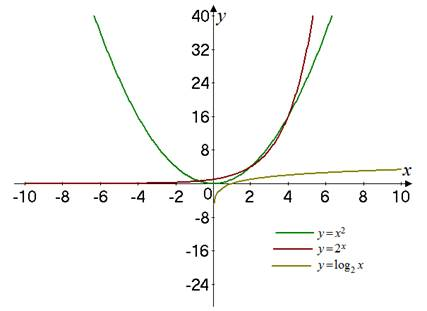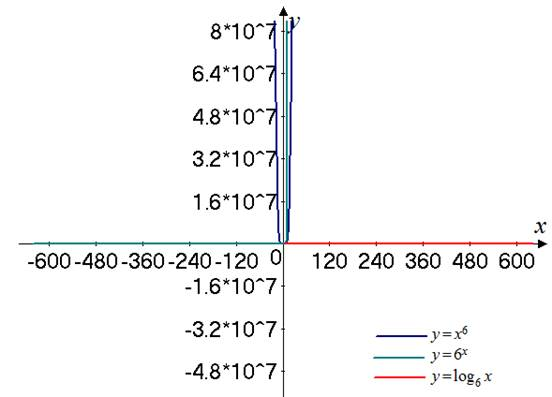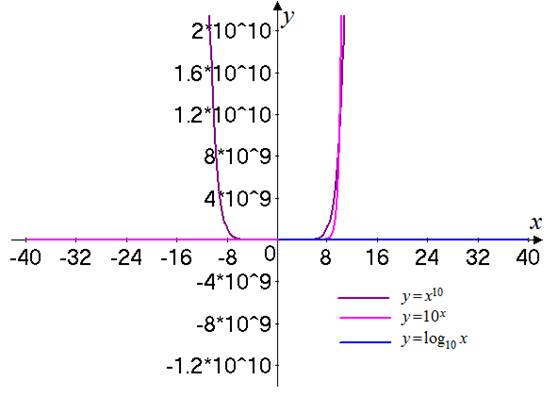# The graph for the given equations on the same screen for the two or three values of a &gt; 1 . Also, find the function that has the largest values and smallest value for the for x .### Single Variable Calculus: Concepts...

4th Edition
James Stewart
Publisher: Cengage Learning
ISBN: 9781337687805### Single Variable Calculus: Concepts...

4th Edition
James Stewart
Publisher: Cengage Learning
ISBN: 9781337687805

#### Solutions

Chapter 1, Problem 30RE
To determine

## To find: The graph for the given equations on the same screen for the two or three values of a>1 . Also, find the function that has the largest values and smallest value for the for x .

Expert Solution

The graph for the given functions is shown in Figure 1, Figure 2 and Figure 3 and the largest value is of the function y=ax and the function has the smallest value for large value of x .

### Explanation of Solution

Given:

The given function are,

y=axy=xay=logax

Calculation:

Consider the given functions,

y=axy=xay=logax

Consider the case when a=2 .

The graph for all the function is shown in Figure 1Figure 1

Consider the case when a=6 .

The graph for all the function is shown in Figure 2Figure 2

Consider the case when a=10 .

The graph for all the function is shown in Figure 3Figure 3

From the graph in Figure 1, 2, and 3 it is observed that the function y=ax has the largest values and y=logax has the smallest value for the larger values of x .

### Have a homework question?

Subscribe to bartleby learn! Ask subject matter experts 30 homework questions each month. Plus, you’ll have access to millions of step-by-step textbook answers!